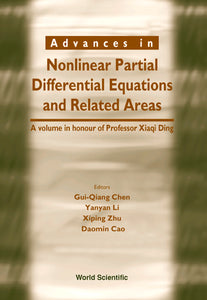# Advances In Nonlinear Partial Differential Equations And Related Areas: A Volume In Honor Of Prof Xia

Gui-qiang Chen

Format: Print Book

ISBN: 9789810236649

• \$164.70
Unit price per
Tax included.

This volume is a collection of research papers on nonlinear partial differential equations and related areas, representing many aspects of the most recent developments in these important areas. In particular, the following are included: nonlinear conservation laws, semilinear elliptic equations, nonlinear hyperbolic equations, nonlinear parabolic equations, singular limit problems, and analysis of exact and numerical solutions. Important areas such as numerical analysis, relaxation theory, multiphase theory, kinetic theory, combustion theory, dynamical systems, and quantum field theory are also covered.

Format: Hardcover
No of Pages: 448
Imprint: World Scientific
Publication date: 19981207